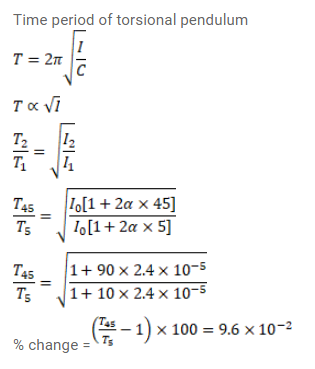# A torsional pendulum consists of a solid disc connectedQuestion:

A torsional pendulum consists of a solid disc connected to a thin wire $\left(\alpha=2.4 \times 10^{-5} /{ }^{\circ} \mathrm{C}\right)$ at its center. Find the percentage change in the time period between peak winter $\left(5^{\circ} \mathrm{C}\right)$ and peak summer $\left(45^{\circ} \mathrm{C}\right)$.

Solution: